### Home > CALC > Chapter 10 > Lesson 10.4.1 > Problem10-138

10-138.
1. Examine the integrals below. Consider the multiple tools you have available for integrating and use the best one. After evaluating each integral, write a short description of the method you used. Homework Help ✎

1.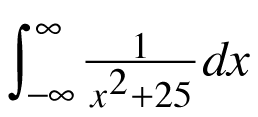2.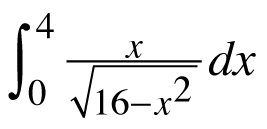3.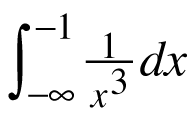4.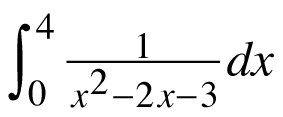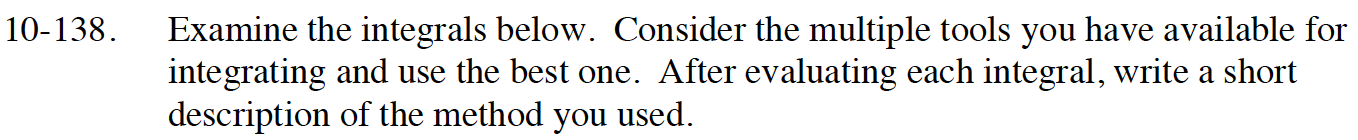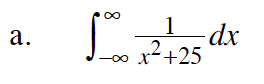$=\frac{1}{25}\int_{-\infty}^{\infty}\frac{1}{(x/5)^2+1}dx$

$=\lim_{a\to-\infty}\frac{1}{25}\int_{a}^{0}\frac{1}{(x/5)^2+1}dx+\lim_{b\to\infty}\frac{1}{25}\int_{0}^{b}\frac{1}{(x/5)^2+1}dx$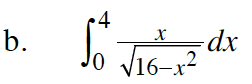Use substitution. Let u = 16 – x^2.

A limit is needed to evaluate this integral.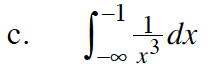$=\lim_{c\to-\infty}\int_{c}^{-1}x^{-3}dx$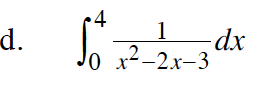$\frac{1}{x^2-2x-3}=\frac{-1/4}{x+1}+\frac{1/4}{x-3}$